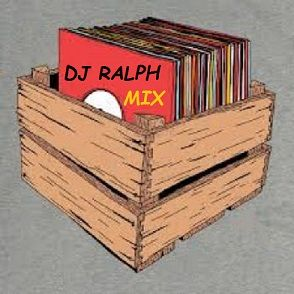[ Post a Response | Ultimate Crave Message Board ]

)))))))))))))))))))))))))))))))Whatcha!..."Member? You 'Member! ((((((((((((((((((((((((((((((Whatcha!..."Member? You 'Member!...I'm bringing it back for you by special request Click on The Link ---> https://soundcloud.com/dj-ralph-of-east-l-a/holidays-gift-mixMessage Thread )))))))))))))))))))))))))))))))Whatcha!..."Member? You 'Member! (((((((((((((((((((((((((((((( - DJ RALPH E.L.A. March 14, 2015, 9:59 pm« Back to index

Post a Response